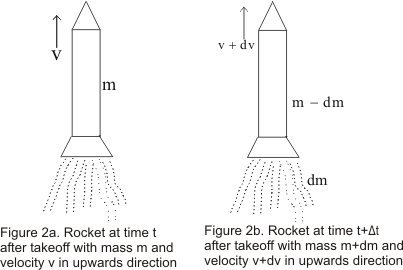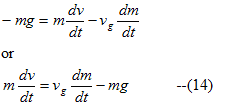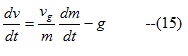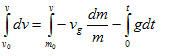# Rocket propulsion system

## (5) Motion of the system with varying mass(Rocket)

• Up till now while studying classical mechanics we have always considered the particle under consideration to have constant mas
• Some times it is required to deal with the particles or system of particles in which mass is varying and motion of the rocket is one such examples
• In a rocket fuel is burned and the exhaust gas is expelled out from the rear of the rocket
• The force exerted by the exhaust gas on the rocket is equal and opposite to the force exerted by the rocket to expel it
• This force exerted by the exhaust gas on the rocket propels the rocket forwards
• The more gas is ejected from the rocket ,the mass of the rocket decreases• To analyse this process let us consider a rocket being fired in upwards direction and we neglect the resistance offered by the air to the motion of the rocket and variation in the value of the acceleration due to gravity with height
• Figure above shows a rocket of mass m at a time t after its take off moving with velocity v.Thus at time t momentum of the rocket is equal to mv.THus
$p_i=mv$
• Now after a short interval of time dt,gas of total mass dm is ejected from the rocket
• If $v_g$ represents the downward speed of the gas relative to the rocket then velocity of the gas relative to earth is
$v_{ge}=v-v_g$
And its momentum equal to
$dmv_{ge}=dm(v-v_g)$
• At time t+dt,the rocket and unburned fuel has mass m-dm and its moves with the speed v+dv.Thus momentum of thee rocket is
$=(m-dm)(v+dv)$

• Total momentum of the system at time t+dt is
$p_f=dm(v-v_g)+(m-dm)(v+dv)$
Here system constitute the ejected gas and rocket at the time t+dt
• From Impulse momentum relation we know that change in momentum of the system is equal to the product of the resultant external force acting on the system and the time interval during which the force acts
• Here external force on the rocket is weight -mg of the rocket ( the upward direction is taken as positive)
• Now
Impulse=change in momentum
$F_{ext}dt=p_f-p_i$
or
$-mgdt=dm(v-v_g)+(m-dm)(v+dv) - mv$
or
$-mgdt=mdv-v_gdm-dmdv$
term dmdv can be dropped as this product is negligible in comparison of other two terms
Thus we have• In equation (14) dv/dt represent the acceleration of the rocket ,so mdv/dt =resultant force on the rocket

Therefore
Resultant Force on rocket=Up-thrust on the rocket - weight of the rocket
where up-thrust on rocket=vg (dm/dt)
• The up-thrust on rocket is proportional to both the Relative Velocity (vg) of the ejected gas and the mass of the gas ejected per unit time (dm/dt)
• Again from equation (14)As rocket goes higher and higher ,value of the acceleration due to gravity g decreases continuously .The values of vg and dm/dt practically remains constant while fuel is being consumed but remaining mass m decreases continuously .This result in continuous increase in acceleration of rocket until all the fuel is burned up

• Now we will find the relation between the velocity at any time t and remaining mass.Again from equation (15) we have
$dv=v_g \frac {dm}{m} -gdt$

• Here dm is a +ve quantity representing mass ejected in time dt.So change in mass of the rocket in time dt is -dm.So while calculating total mass change in rocket,we must change the sign of the term containing dm

$dv=-v_g \frac {dm}{m} -gdt$                 --(16)
• Initially at time t=0 if the mass and velocity of the rocket are m0 and v0 respectively.After time t if m and v are mass and velocity of the rocket then integrating equation (16) within these limitsOn evaluating this integral we get
$v-v_0=-v_g(ln m- ln m_0)-g(t-0)$
or $v=v_0+v_g ln \frac {m_0}{m} -gt$                (17)
• equation(17) gives the change in velocity of the rocket in terms of exhaust speed and ration of initial and final masses at any time t
• The speed acquired by the rocket when the whole of the fuel is burned out is called burn-out speed of the rocket